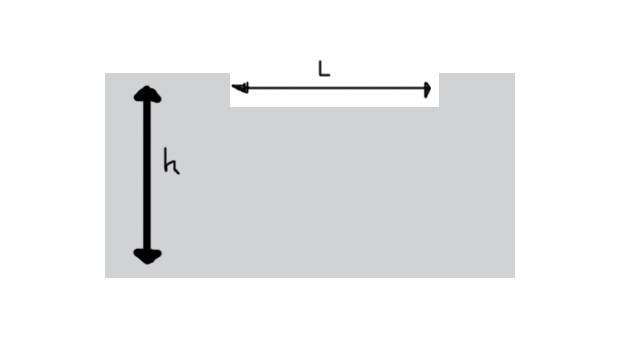# Qualitative description of unbounded ocean system

## Homework Statement

[/B]
a) Suppose that you have the unbounded ocean show in the picture, where $h = 2000$ m. At $t = 0$, the system is allowed to relax. Make a qualitative description of its evolution and final state if i) $L = 100$ m, and ii) $L = 10,000$ m.b) At what speed should an observer move in order to observe the same wavelength of a wave packet traveling over a basin of constant depth?

## Homework Equations

None, because this is a qualitative question? I guess $c_g = \frac{\partial \omega}{\partial k}$ may be useful for part b.

## The Attempt at a Solution

For part a.i, because $L$ is small, is seems as though what will happen is the higher sides of that rectangular space in the ocean will come crashing towards each other, then hit, then they'll sort of rebound back out , and eventually the ocean will settle back into a still surface. For part a.ii., because $L$ is bigger, the water from each higher side will take longer to reach each other, but I'm not sure how else that would change the situation, and I have no idea what $h$ has to do with this whole situation at all, really.

For part b), I am confused on the wording of this part. When it says in order to observe the same wavelength of a wave packet, what is the "same" referring to? The observer observes the same wavelength as...?

I'm sorry for how messy my answers here are. I guess I really do have a hard time with qualitative questions like this. I would really appreciate any help!

## Answers and Replies

Bystander
Science Advisor
Homework Helper
Gold Member
"Wavelength" is the length of the displaced surface.